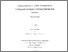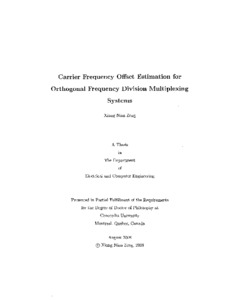Title:

# Carrier frequency offset estimation for orthogonal frequency division multiplexing systems

Zeng, Xiang Nian (2008) Carrier frequency offset estimation for orthogonal frequency division multiplexing systems. PhD thesis, Concordia University.Preview
Text (application/pdf)
NR45685.pdf - Accepted Version
2MB

## Abstract

Orthogonal frequency division multiplexing (OFDM) is an attractive modulation scheme used in wideband communications because it essentially transforms the frequency selective channel into a flat fading channel. Furthermore, the combination of multiple-input multiple-output (MIMO) signal processing and OFDM seems to be an ideal solution for supporting reliable high data rate transmission for future wireless communication systems. However, despite the great advantages OFDM systems offer, such systems present challenges of their own. One of the most important challenges is carrier frequency offset (CFO) estimation, which is crucial in building reliable wireless communication systems. In this thesis, we consider CFO estimation for the downlink and uplink OFDM systems. For the downlink channel, we focus on blind schemes where the cost functions are designed such that they exploit implicit properties associated with the transmitted signal where no training signal is required. By taking the unconditional maximum likelihood approach, we propose a virtual subcarrier based blind scheme for MIMO-OFDM systems in the presence of spatial correlation. We conclude that the presence of spatial correlation does not impact the CFO estimation significantly. We also propose a CFO estimator for OFDM systems with constant modulus signaling and extend it to MIMO-OFDM systems employing orthogonal space-time block coding. The curve fitting method is used which gives a closed-form expression for CFO estimation. Therefore, the proposed scheme provides an excellent trade-off between complexity and performance as compared to prominent existing estimation schemes. Furthermore, we design a blind CFO estimation scheme for differentially modulated OFDM systems based on the finite alphabet constraint. It can achieve better performance at high signal-to-noise ratios (SNRs) at the expense of some additional computational complexity as compared to the schemes based on the constant modulus constraint. The constrained Cramer-Rao lower bound (CRLB) is also derived for the blind estimation scheme. As for the uplink channel, which is a more challenging problem, we propose two training aided schemes. One is based on a scalar extended Kalman filter (EKF) and the other one is on the variable projection (VP) algorithm. For both schemes, we assume that the system uses an arbitrary subcarrier assignment scheme, which is more involved than the other two schemes, namely block and interleaved subcarrier assignment scheme. In the first scheme, to apply the scalar EKF algorithm, we represent the measurement equation as a function of a scalar state, i.e., each user's CFO, in lieu of a state vector which consists of both CFO and channel coefficients by replacing the unknown channel coefficients with a nonlinear function of CFO. This proposed scheme can achieve the CRLB at high SNR for two users with a complexity lower than that of the alternating-projection method. In the second scheme, the VP algorithm is used for CFO estimation which is followed with a robust minimum mean square error (MMSE) estimator for channel estimation. In the VP algorithm, the nonlinear least square cost function is optimized numerically by updating the CFOs and channel coefficients separately at each iteration. We demonstrate that this proposed scheme is superior to the existing methods in terms of convergence speed, computational complexity and estimation performance.

Divisions: Concordia University > Gina Cody School of Engineering and Computer Science > Electrical and Computer Engineering Thesis (PhD) Zeng, Xiang Nian xix, 125 leaves : ill. ; 29 cm. Concordia University Ph. D. Electrical and Computer Engineering 2008 Ghrayeb, Ali 975192 Concordia University Library 22 Jan 2016 17:58 18 Jan 2018 17:39 http://clues.concordia.ca/search/c?SEARC...
All items in Spectrum are protected by copyright, with all rights reserved. The use of items is governed by Spectrum's terms of access.

Repository Staff Only: item control pageResearch related to the current document (at the CORE website)
Back to top